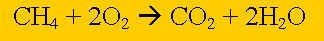Mass-mass stoichiometryMethane burns in oxygen to produce carbon dioxide and water according to the equation above. If 27 grams of water are formed calculate the mass of methane used. Step 1 Calculate the number of mole of water present. Step 2 Calculate the ratio from the prefixes in the balanced equation above Step 3 Calculate the number of mole of methane used. Step 4 Calculate the mass of water produced.

Home